# Right View of a Binary Tree

## Introduction

Let’s assume a scenario in which Ninja decides to move his point of vision to the right side of the tree instead of looking from the front of a binary tree. The right view of the binary tree would contain all the nodes present at the right face of a tree.

This means that the nodes to be printed are the rightmost nodes in the tree. We will work on two different approaches to solve this problem.

Let’s understand using an example what would be the right view of a binary tree.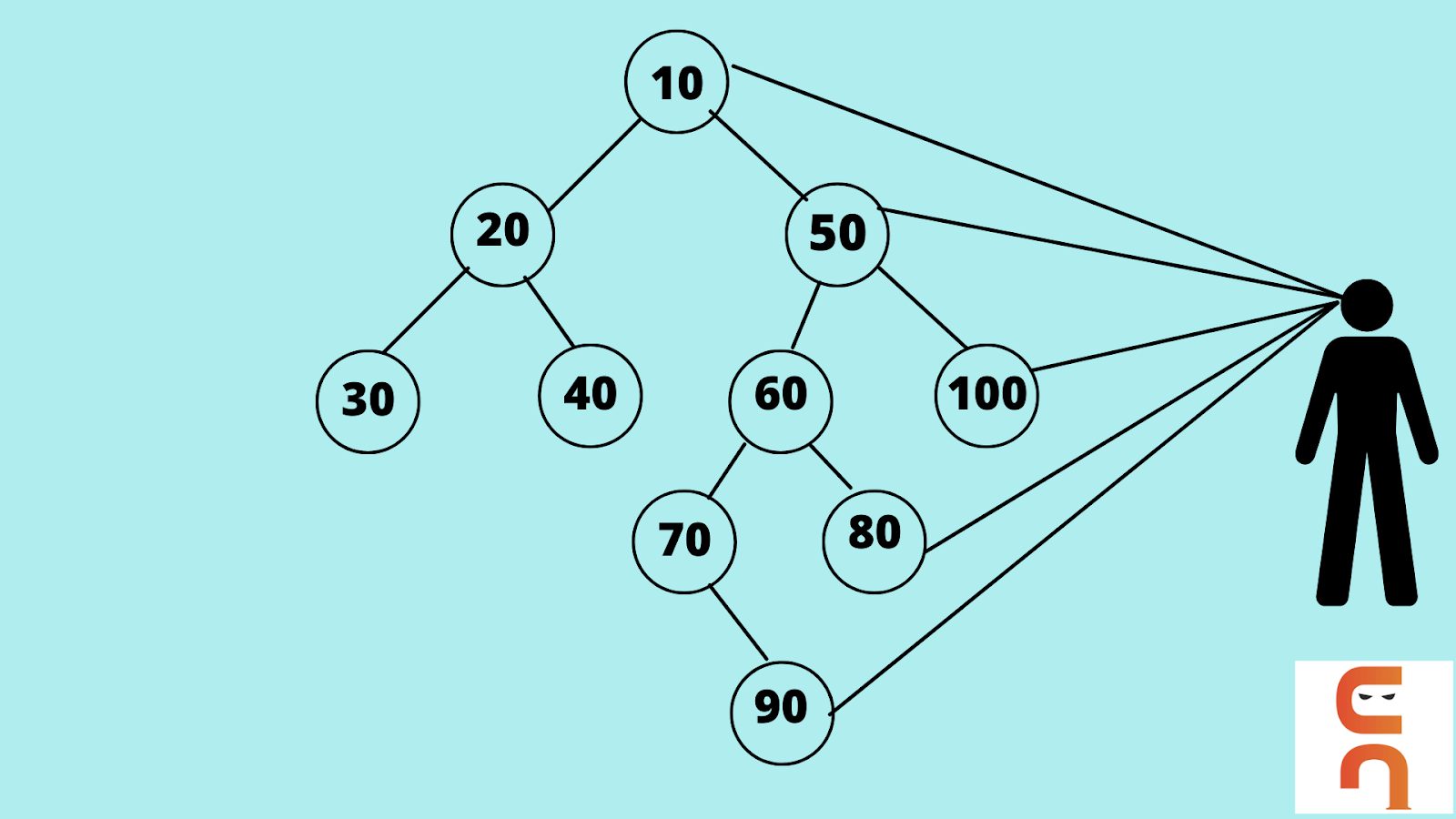Just see in the above example the line of sight in the right direction. The right view of the tree wants the Ninja to observe all the nodes through the right and, in the above example, those visible nodes are - 10, 50, 100, 80, and 90. These nodes block the view of the rest of the nodes when the tree is viewed from the right direction, thus giving us only the rightmost nodes of the tree.

The problem statement is, Given any binary tree print the right view of the binary tree.

We can have two approaches to solve this problem. Let’s understand the concept a bit more before considering the algorithms.

In the above figure, we know that the last node in each level of the tree is the right view of the binary tree.

So instead of traversing the tree from left to right, we traverse each level from right to left.

Let’s have a look at the tree,We can, therefore, say that the first node in this type of traversal is the right view of a binary tree. We have the first node of each level as follows -

Right traversal of the tree:-

Level 0 (root node) - 10

Level 1 - 50

Level 2 - 100

Level 3 - 80

Level 4 - 90

To obtain the above result, we have two types of traversals that can be used to solve the problem of the right view of a binary tree.

1. Recursive Traversal
2. Iterative (Level Order) Traversal

Let’s go through the algorithms to understand the approaches mentioned above.## Recursive Approach

We will be solving the problem using reverse preorder traversal. The general preorder traversal means it follows the below structure -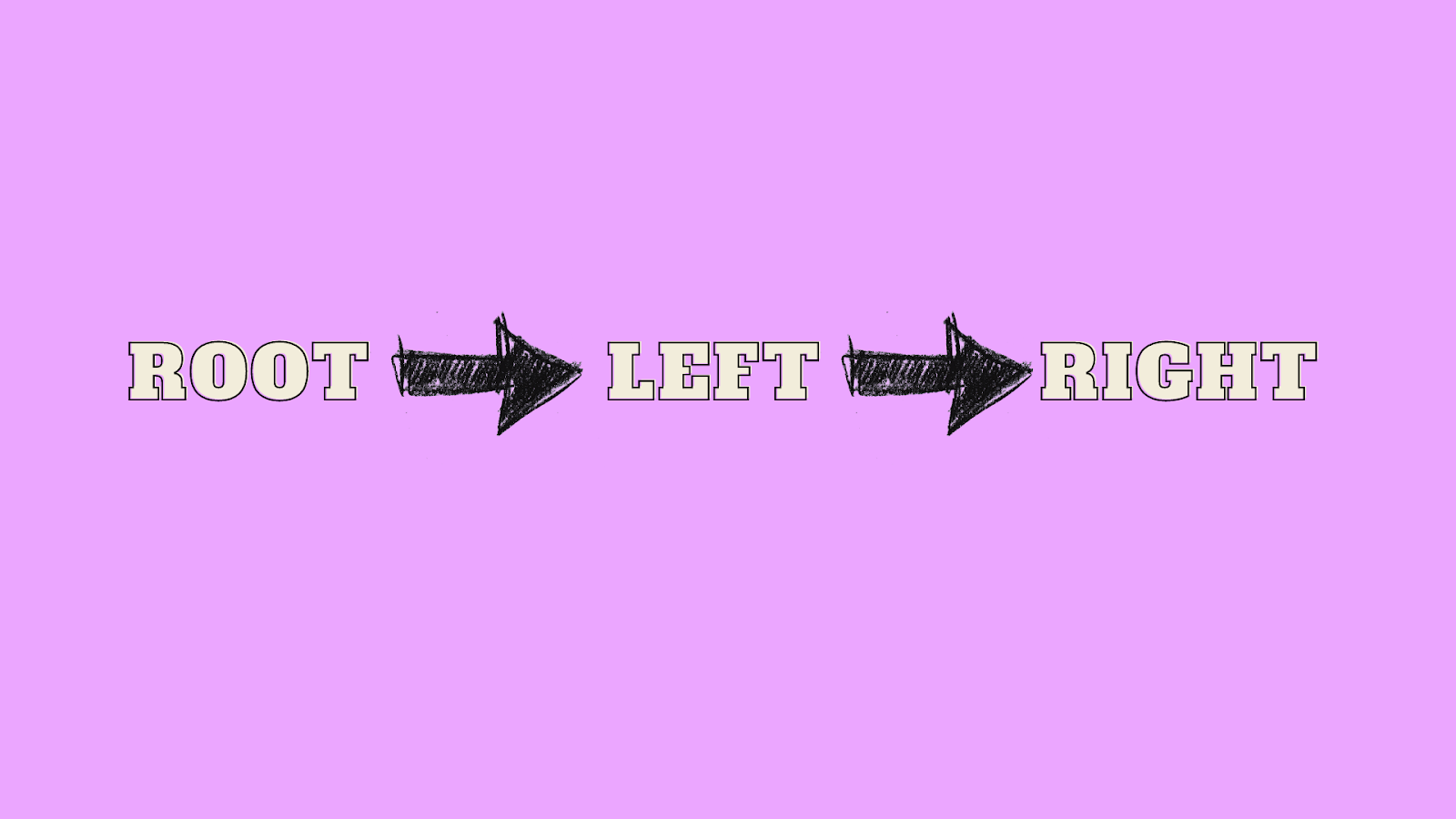But we would reverse the structure because eventually, we only need the first rightmost nodes,

Therefore we will convert the above structure to,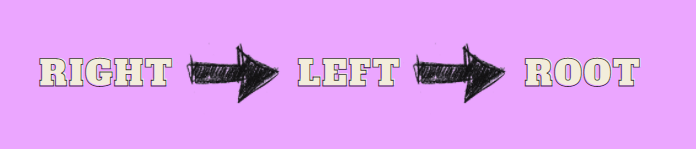The algorithm would use a concept of recursion as the node would pass for all  right children and left children according to the level of the tree.

### Algorithm for Recursive Approach

For this approach to find the right view of a binary tree, we will declare a variable level that we would set to 0. We will print all the nodes which are first to be visited when a new level is encountered in the tree.

We will reverse the general preorder traversal and call right and then call the left child recursively and update the level whenever the function is called.  This approach requires the reversal preorder traversal and the recursion concept to obtain the final result. Let’s go through the algorithm to understand the approach.

``````
//set level = 0
//set maxlevel = 0
level = 0
function RightView(node,level)
{
if(node == null) return
//used to check if the level is traversed
//or is being visited for the first time
if(level == list.size)
{
Print the value of the node
Set maxlevel = level
}

RightView(node->right, level+1)
RightView(node->left, level+1)
}``````

### Implementation in Java

``````import java.util.*;

public class Main {

public static class Node {
int data = 0;
Node left = null;
Node right = null;

Node(int data) {
this.data = data;
}
}

static int idx = 0;
static int max_level = 0;
public static Node create_Tree(int[] arr) {
if (idx == arr.length || arr[idx] == -1) {
idx++;
return null;
}

Node node = new Node(arr[idx++]);

node.left = create_Tree(arr);
node.right = create_Tree(arr);

return node;
}

public static void RightView (Node node, int level) {
if (node == null)
return;

if (max_level < level) {
System.out.print(node.data + " ");
max_level = level;
}
RightView(node.right, level + 1);
RightView(node.left, level + 1);
}

public static void viewSet(Node root) {
RightView(root,1);
}

public static void solve() {
int[] arr = {10,20,30,-1,-1,40,-1,-1,50,60,70,-1,-1,80,90,-1,-1,-1,100,-1,-1 };
System.out.println("Using Recursion Method");
Node root = create_Tree(arr);
viewSet(root);
}

public static void main(String[] args) {
solve();
}
}
``````

Output

``````Using Recursion Method
10 50 100 80 90``````

Time Complexity: The Time Complexity of the above approach to find the right view of a binary tree is O(N), where N is the number of nodes in the binary tree.

Space Complexity: The Space Complexity of the above approach to find the right view of a binary tree is O(H), where H is the height of the binary tree. In case of skewed trees the height of the tree can become N which gives a worst of O(N).

## Iterative Approach

We will solve the problem by using the classic level order traversal and the queue data structure.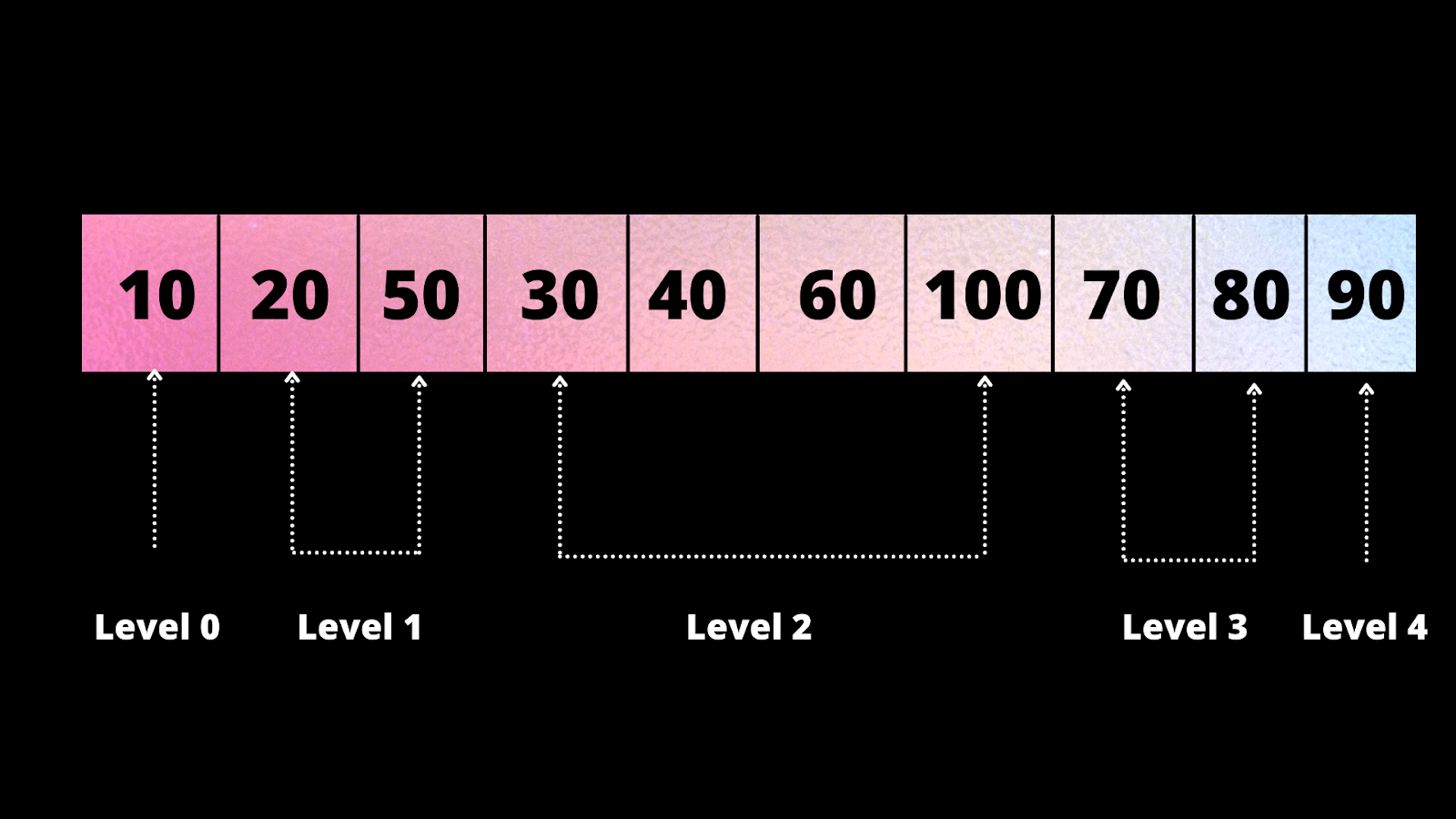So, to perform Level Order Traversal, the nodes would be considered level-wise. This means that each node would access the child at each level before moving to the next level.

We have these nodes respective to each level,

Level 0 - 10

Level 1 - 20, 50

Level 2 - 30, 40, 60, 100

Level 3 - 70, 80

Level 4 - 90

Notice the last node in each level is the node that is to be printed for the result.

### Algorithm for Iterative Approach

In the algorithm, we would do a level order traversal of the tree and would print the last node of each level. Since we will iterate using a for loop for each node in the level this approach is known as an Iterative Approach. We will use a queue as a data structure to store the last nodes for each level. We will be using the poll function of the queue to find the last element of the level. We will check the element’s position if it’s the previous element using the size of the queue. Now let us go through the algorithm for an iterative approach that is used to display the right view of a binary tree.

``````enqueue root
while queue is not empty
{
// find the length of the queue
int size = queue.size()
// run a loop from 0 to size
for(i =0, i<size, i++)
{
dequeue root
//check if the element is present at last
if(i==size-1)
{
print node.data
}
enqueue left child
enqueue right child
}
}``````

### Implementation in Java

``````
import java.util.*;

public class Main {

public static class Node {
int data = 0;
Node left = null;
Node right = null;

Node(int data) {
this.data = data;
}
}

static int idx = 0;

public static Node create_Tree(int[] arr) {
if (idx == arr.length || arr[idx] == -1) {
idx++;
return null;
}

Node node = new Node(arr[idx++]);

node.left = create_Tree(arr);
node.right = create_Tree(arr);

return node;
}

public static void RightView(Node node) {

while (que.size() != 0) {

int size = que.size();
for(int i =0;i<size;i++) {
Node n = que.poll();

if(i== size-1) {
System.out.print(n.data + " ");
}
if(n.left !=null) {
}
if(n.right != null) {
}
}
}
}

public static void solve() {
int [] arr ={10,20,30,-1,-1,40,-1,-1,50,60,70,-1,-1,80,90,-1,-1,-1,100,-1,-1 };
Node root = create_Tree(arr);
System.out.println("Using Iterative Approach");
RightView(root);
}

public static void main(String[] args) {
solve();
}
}``````

Output

``````
Using Iterative Approach
10 50 100 80 90``````

Time Complexity: The Time complexity of the Iterative Approach to find the right view of a binary tree is O(N), where N is the number of nodes in the tree.

Space Complexity: The Space Complexity of the Iterative Approach to find the right view of a binary tree is O(N), where N is the number of nodes in the tree.

1. What is the Right view of a Binary Tree?
The right view of a binary tree means to print all the nodes visible when the tree is seen from the right direction. The final output would have the nodes, which are the most rightmost in a tree, as these nodes hide the rest of the nodes.
When a person limits his line of sight in a single direction, only nodes visible in that area would be visible to him. The right view of a Binary Tree consists of nodes which are the rightmost nodes in a tree.

2. Which approach is better to find the right view of a binary tree?
The recursive approach is better than the iterative approach as a limited space of O(H) is used in the algorithm, where H is the tree’s height. Whereas the Iterative algorithm uses level order traversal, the worst case in a level order traversal is a lot more than the recursive approach.

3. What is a right-skewed binary tree?
A right-skewed binary tree in which all the nodes have the right child or no child at all. A right-skewed binary tree is also known as a right dominating tree. The below image is an example of a Right skewed Binary Tree.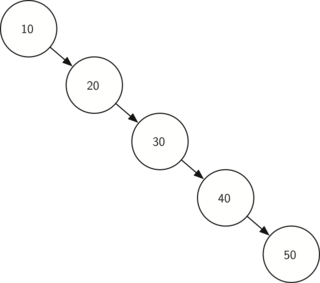4. How will you calculate the space-time complexity for a skewed binary tree for a recursive approach?
The time complexity of the recursive approach would be O(N) where N is the size of the tree.
In this case, instead of a general binary tree, we are given a skewed binary tree therefore, the tree’s height would be the same as the size of the tree as there are no elements in the left section of the binary tree. Hence, in this case, the Space complexity would be O(N) in the recursive approach.

## Key Takeaways

In this blog, we learned how to find the right view of a binary tree and how we can use different approaches to solve this problem.

• In the first approach, we used recursion and reverse preorder traversal to find the right view of a binary tree. We declared a variable level that would be set to 0. We would then print all the nodes which are first to be visited when a new level is encountered in the tree. To do so, we would reverse the general preorder traversal and call right and then call the left child recursively and update the level whenever the function.  This approach requires the reversal preorder traversal and the recursion concept to obtain the final result.

• In the second approach, we used a level order traversal and a queue to solve the problem. We declared a queue that would be used to iterate through each level and find the last node for each level, which we would eventually check with the size of the queue, and if the element is placed at the last of that particular level, we will print the node. This would be called for the left child nodes and the right child nodes. The two key concepts involved in this approach are level order traversal and Queues.

If you want to learn about finding the left view of a binary tree, we have a separate article that covers the approaches used to solve the problem. Do give it a read.

Visit here to learn more about trees. And practice similar problems on CodeStudio. If you liked this blog, share it with your friends.